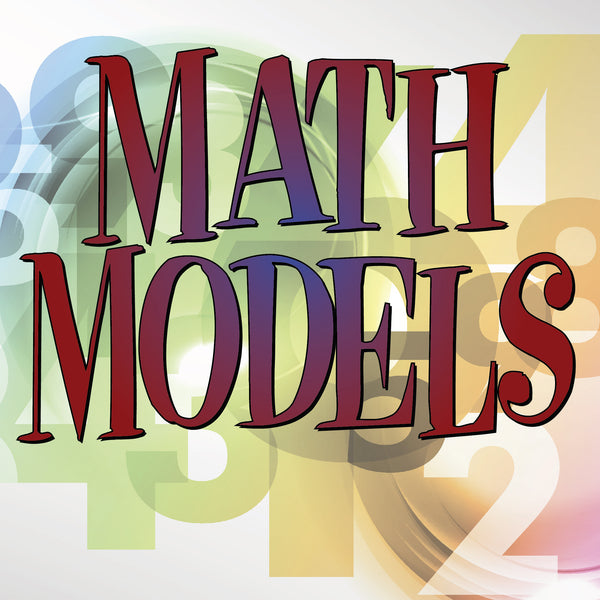Math ModelsStudents build on K-8 and Algebra I foundations using algebraic, graphical, and geometric reasoning to recognize patterns and structure, to model information and to solve problems from various fields of study. Students will use mathematical methods to model and solve real-life applied problems involving money, data, chance, patterns, music design, and science. Mathematical models from algebra, geometry, probability, and statistics will be used to solve problems in mathematical and nonmathematical situations. Justification, proof, and computation will also be used in problem-solving.

Regular price \$299.95 Sale